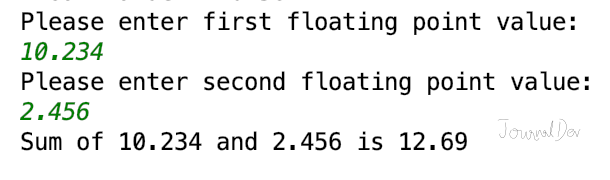# Python Convert String to float

Published on August 3, 2022By Pankaj
Developer and author at DigitalOcean.While we believe that this content benefits our community, we have not yet thoroughly reviewed it. If you have any suggestions for improvements, please let us know by clicking the “report an issue“ button at the bottom of the tutorial.

We can convert a string to float in Python using the `float()` function. This is a built-in function used to convert an object to a floating point number. Internally, the float() function calls specified object __float__() function.

## Python Convert String to float

Let’s look at a simple example to convert a string to float in Python.

``````s = '10.5674'

f = float(s)

print(type(f))
print('Float Value =', f)
``````

Output:

``````<class 'float'>
Float Value = 10.5674
``````

### Why do we need to convert a string to float?

If we are getting float value from user input through the terminal or reading it from a file, then they are string objects. We have to explicitly convert them to float so that we can perform necessary operations on it, such as addition, multiplication, etc.

``````input_1 = input('Please enter first floating point value:\n')
input_1 = float(input_1)

input_2 = input('Please enter second floating point value:\n')
input_2 = float(input_2)

print(f'Sum of {input_1} and {input_2} is {input_1+input_2}')
``````Ideally, we should use `try-except` block to catch exceptions in case of invalid input from user. If you are not familiar with string formatting using f prefix, please read f-strings in Python.

## Python Convert float to String

We can convert float to a string easily using str() function. This might be required sometimes where we want to concatenate float values. Let’s look at a simple example.

``````f1 = 10.23
f2 = 20.34
f3 = 30.45

# using f-string from Python 3.6+, change to format() for older versions
print(f'Concatenation of {f1} and {f2} is {str(f1) + str(f2)}')
print(f'CSV from {f1}, {f2} and {f3}:\n{str(f1)},{str(f2)},{str(f3)}')
print(f'CSV from {f1}, {f2} and {f3}:\n{", ".join([str(f1),str(f2),str(f3)])}')
``````

Output:

``````Concatenation of 10.23 and 20.34 is 10.2320.34
CSV from 10.23, 20.34 and 30.45:
10.23,20.34,30.45
CSV from 10.23, 20.34 and 30.45:
10.23, 20.34, 30.45
``````

If we don’t convert float to string in the above program, the `join()` function will throw an exception. Also, we won’t be able to use + operator to concatenate as it will add the floating point numbers.

You can checkout complete python script and more Python examples from our GitHub Repository.

Reference: float() official documentationPankaj

author

Developer and author at DigitalOcean.